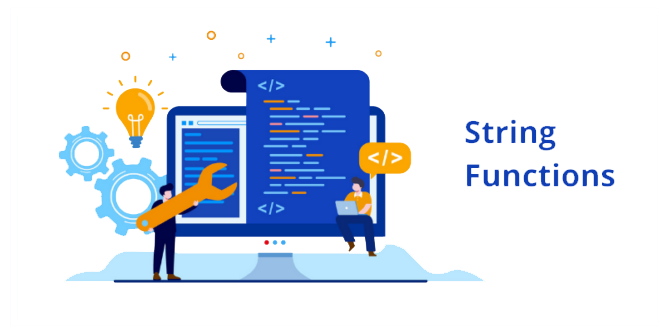Decimal To Binary Converter

Enter the decimal number to decode, and then click "Convert!":

The number in binary representation:

Need to convert binary to decimal?

How Do You Use the Decimal to Binary Converter Tool?

To use the Decimal to Binary converter, simply enter your decimal value you would like to convert in the first text box, then click 'convert!'. The tool will then display the converted binary value in the text box directly below. From there, you can use the decimal to binary converter tool as many times as you like!

Why Might You Need the Decimal to Binary Converter Tool?

The binary system is one of the most simple numerical systems as it is only made up of 2 symbols, 0 and 1. While the numeric system dates back to the 17th century, the binary system is commonly used today in modern computers. The two symbols represent the states, on or off, which allows the computer to understand the data. Programmers are very familiar with converting binary to decimal numbers (or vise versa) as it is a common necessity in their line of work. There are two common ways to convert binary to decimal, one being 'Positional Notation' and the other, 'Doubling'. The easiest method, is of course, using a decimal to binary online converter tool such as what String-Functions offers.

Keywords: binary decode a string text, tool, on line tool, tech career decoder.